# Discount Rate

What is a Discount Rate?

The discount rate refers to a value used in DCF (Discounted Cash Flow) analysis to adjust the future value of earnings into present-day dollars. For the interest rate set by the Federal Reserve, see Federal Discount Rate.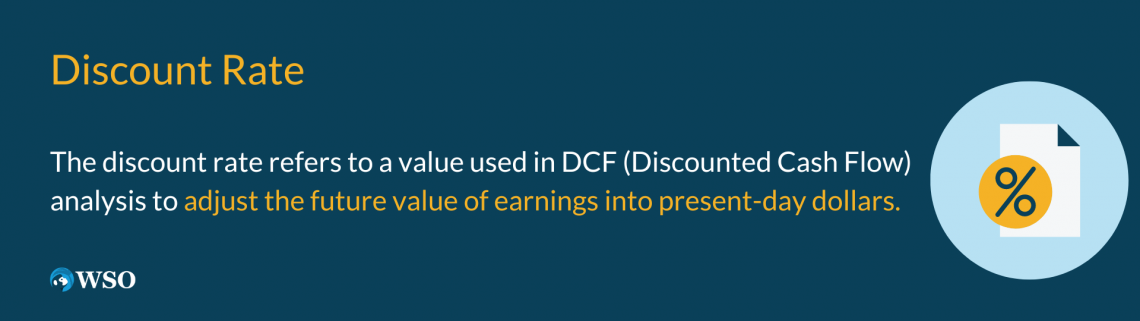Contrary to what you might think, the discount rate does not represent that 15% off label on your favorite brand of cheese-filled danishes.

Instead, the one we're talking about applies to businesses and investments, where it helps investors determine the profitability of a planned project. Depending on the type of investment, the value used will vary.

DCF analysis helps us compare the invested inputs into a project with the predicted outputs it will generate. However, the time value of money varies between the inputs, which are paid today, and the outputs, which are to be gained in the future.

To resolve this discrepancy, the discount rate is used to find the present value of future cash flows. In other words, it allows you to solve for the value of a sum of money in the future in today's terms.

Let's compare it to compound interest, which is its inverse. For example, take a savings account that earns 5% a year, compounded annually. If it has a principal of \$1,000, here's how much it would have in ten years:

1000 x (1.05)10 = 1628.89

More generally, this formula can be simplified to:

P(1 + (r/n))nt = A

Where

• P is principal, also known as the initial amount
• r is the interest rate
• b is the number of times the interest is applied per period, e.g., four times if it was quarterly
• t is the number of periods, typically in years
• A is the resulting amount

Conversely, you can find the discount rate if you have the future and the present value:

(1628.89/1000)0.1 - 1 = 0.05

Here is the more general formula: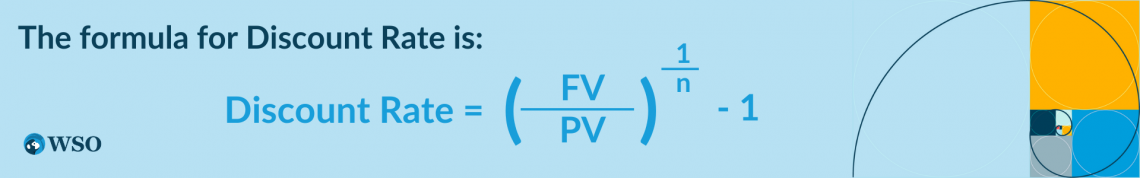Where

• FV stands for future value
• PV stands for the present value
• n is the number of times interest is applied per period
Key Takeaways

• The discount rate is a measure used in the discounted cash flow analysis to adjust the stream of future earnings to account for the time value of money.
• Higher values imply that the present value of the future earnings is worth less.
• Decision makers compare the discounted value of the cash flow to the investment cost to determine whether an investment or project is viable.
• There are five values commonly used as the discount rate:
• The weighted average cost of capital
• Risk-free rate
• Hurdle rate
• Cost of equity
• Cost of debt
• Changes in these values over time imply that the model will never be a precise calculation. Instead, it is more of an estimate.

## What is the discount rate used for?

It is used to find the present value of future free cash flows in DCF analysis. Depending on the project being evaluated, the value used can vary.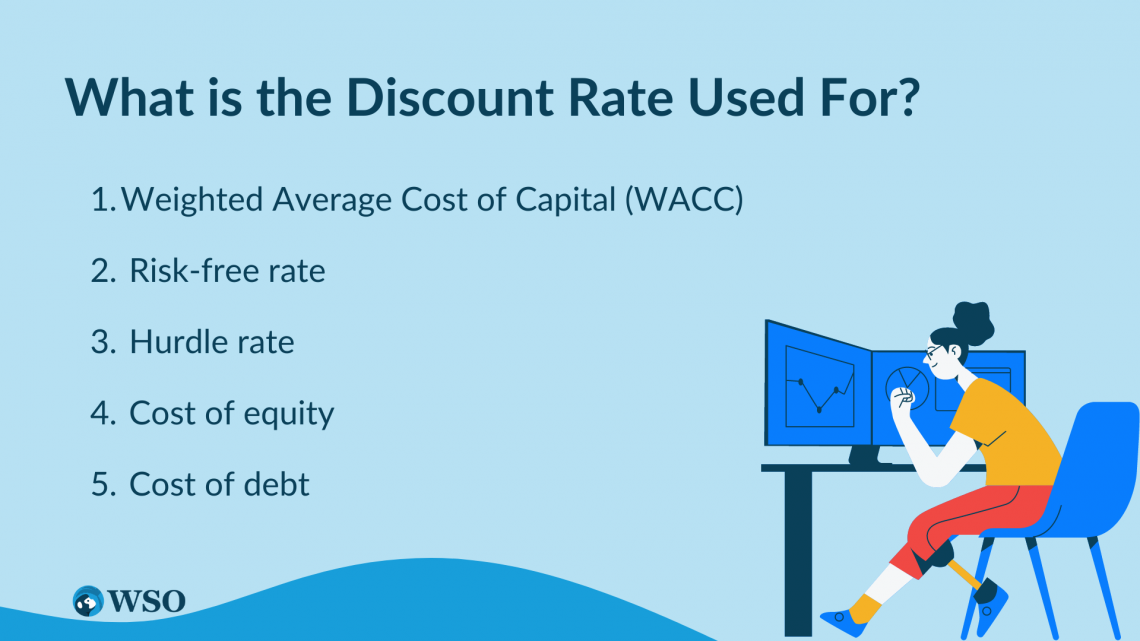Let's look at some commonly used values:

1. Weighted average cost of capital (WACC)

The WACC uses a company's capital structure to balance the weighted average between the cost of equity and the cost of debt. This value measures the average cost for a company when attracting monetary capital.

The equity portion of the calculation reflects the demand from shareholders for a sufficient return on their investment, while the debt portion represents the bondholders' demand.

The overall value measures the amount of return both parties require to supply the company with capital. To calculate this value, follow the formula below:Where

• E is the market value of the company's equity
• D is the market value of the company's debt
• V is the sum of E and D
• Re is the cost of equity
• Rd is the cost of debt
• Tc is the corporate tax rate

While other components of the formula are fairly straightforward, the cost of equity and the cost of debt require further discussion. The former measures the amount of return that the company must issue to incentivize investors to buy shares of its stock.

However, stocks are inherently unpredictable. As a result, this value is often estimated through the CAPM (Capital asset pricing model).

In contrast, the cost of debt is simply the amount that the company must pay for its debt financing, specifically through bonds.

The WACC is typically used as the discount rate by investors, who expect a return equal to the WACC when investing.

2. Risk-free rate

The risk-free rate corresponds to the rate of return on an asset with zero risk. Although zero risk is unattainable, a fair approximation typically used for this value is the three-month U.S. Treasury bill.

Note

Even this Treasury bill holds risk, however, as there is always the chance of a government default.

The risk-free rate can be adjusted to match the time frame of the planned project for greater accuracy. For example, if you are using the discounted cash flow model to determine the viability of a ten-year project, then you should use a ten-year Treasury bill as the risk-free rate.

3. Hurdle rate

A hurdle rate is a broader term that refers to the minimum return on a project to make it viable. Think of it as a hurdle-only if the company can earn a specified level of compensation could it move forward with the project.

The hurdle rate reflects several considerations, such as the cost of the project, its relative riskiness, and potential value compared to other projects.

Note

To determine if the project's cost makes it worthwhile, companies use discounted cash flow analysis to calculate the NPV (net present value) while using the hurdle rate as the discount rate.

The risk of the project is also factored into the hurdle rate. When the project bears more risk, the hurdle rate rises to compensate for the increased risk. This risk premium can mean the difference between an undertaken project and one that is not.

Finally, if there are alternative projects that could yield the same return, the hurdle rate reflects the difference in execution costs. If one project has a higher net present value of future cash flows, it would be chosen over the others.

4. Cost of equity

The cost of equity was previously discussed in the WACC, but it can also stand alone as the discount rate. So, for example, companies solely financed by equity would use this value instead.

Note

For investors, the cost of equity represents a value that will rightfully compensate them for undertaking additional risk by investing in a specific company.

Under the CAPM model, the formula for calculating the cost of equity follows:

E(Ri) = Rf + 𝛽i(E(Rm) - Rf)

Where

• E(Ri) is the expected return on the asset, also the cost of equity
• Rf is the risk-free rate of return
• 𝛽i is the beta, representing the volatility of the market
• E(Rm) is the expected market return

The cost of equity evaluates an asset's viability by calculating the expected return of an asset from its volatility and expected market returns. As a discount rate, this value uses the volatility of an asset to adjust for the present value of future cash flows.

5. Cost of debt

The cost of debt was also discussed previously, and just like the cost of equity, it can function independently. Specifically, this term refers to the interest payments on a company's total debt from debt financing.

Companies heavily funded by debt will have a higher cost of debt, which can drag down the DCF-adjusted returns.

## What is the Net Present Value?

The net present value is an important concept closely related to the discount rate. In discounted cash flow analysis, the net present value is the ultimate product that determines whether the project is worthwhile.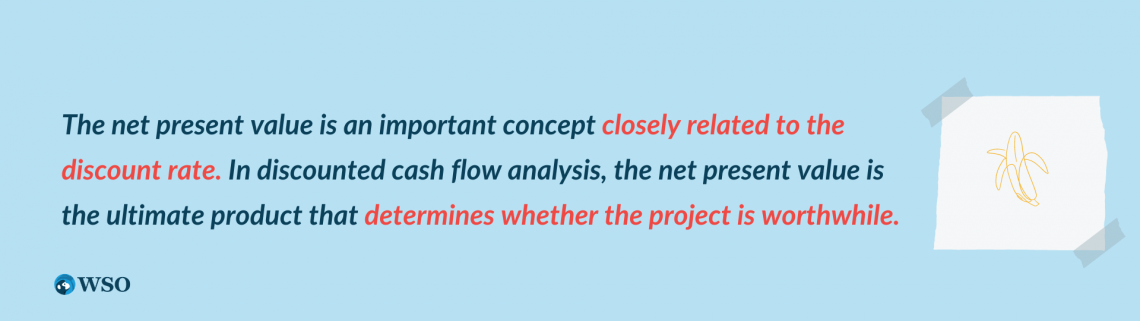To find the net present value, or NPV, use the following formula:

NPV = F/(1+r)n

Where

• F stands for the expected future cash flow in year n
• r stands for the discount rate
• n is the number of years in the future

The NPV adjusts for the time value of money through the assumption that money today is more valuable than money tomorrow as long as there is a positive interest rate. It solves the value of future money in today's terms by reversing the growth from interest.

If the NPV is positive, there is potential for profit because the present value of future cash flows exceeds the current costs.

If the NPV is negative, the project will not be undertaken because it would not be profitable. And when this value equals zero, the company breaks even on its investment.

When the concept of future cash flow is applied to businesses that will continue to generate revenue year after year, the result is a sum of all future revenue, which can then be adjusted to find the NPV.

Take a look at the following formula: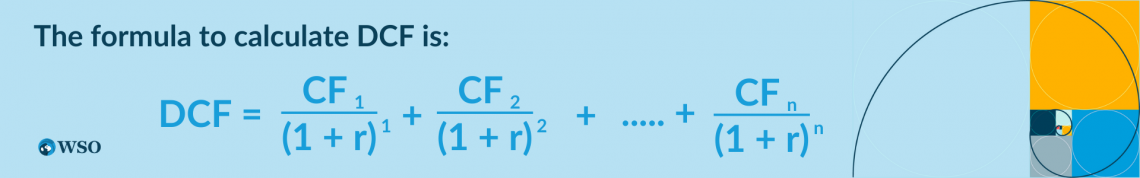Where CFn refers to the cash flow in year n

Instead of finding the NPV for a single year, this formula allows you to find the sum of all future cash flows while adjusting it for time value using a single discount rate. Let's examine the implications provided that this value remains constant.

Over time, the denominator in each fraction grows larger as time eats away at the present value. In the future, the cash flow of most companies will rise. However, compared to the linear growth of this numerator, the denominator grows exponentially.

As you go further and further out into the future, you will find that the present value will become negligible. Think of it as the opposite of compound interest. Instead of having money grow exponentially, the present value decays increasingly faster.

## Issues with the Discount Rate

This acts as an abstraction of what is ultimately much larger and more fluid. In DCF analysis, this value is set to current predictions of the future.This creates an issue, however, because this value can fluctuate over time as the inputs to its calculations change. This is why DCF analysis can become more and more inaccurate as the time frame grows longer.

In addition, the beta used to calculate the cost of equity is based on historical data. Therefore, beta calculations can differ, even with the same formulas, depending on your chosen time frame. For example, a calculation involving years of data will yield a different beta from one involving months.

Furthermore, betas can fluctuate drastically over time. As a result, throughout the DCF analysis, the beta of a company's stock can change dramatically from what it was initially calculated as. This can alter the resulting calculations and whether the project will be profitable.

This is why it is important to acknowledge that the discount rate is ultimately an estimate, an abstraction of the larger mechanisms at work. Therefore, when using it to evaluate whether an investment is worthwhile, for example, be sure to confirm your results with other methods.

When buying a stock, you can verify your conclusion by evaluating other aspects of the company, such as its financial stability and growth potential. Then, using various metrics and techniques, you can protect yourself from avoidable losses.

In other situations, you can hedge against the volatility of the real world by incorporating risk premiums into your calculations. Using risk when finding the discount rate allows you to account for potential losses and compare the relative riskiness of different options.

Note

The discount rate is imperfect, but it can still provide valuable quantitative guidance for companies and investors when making decisions that matter.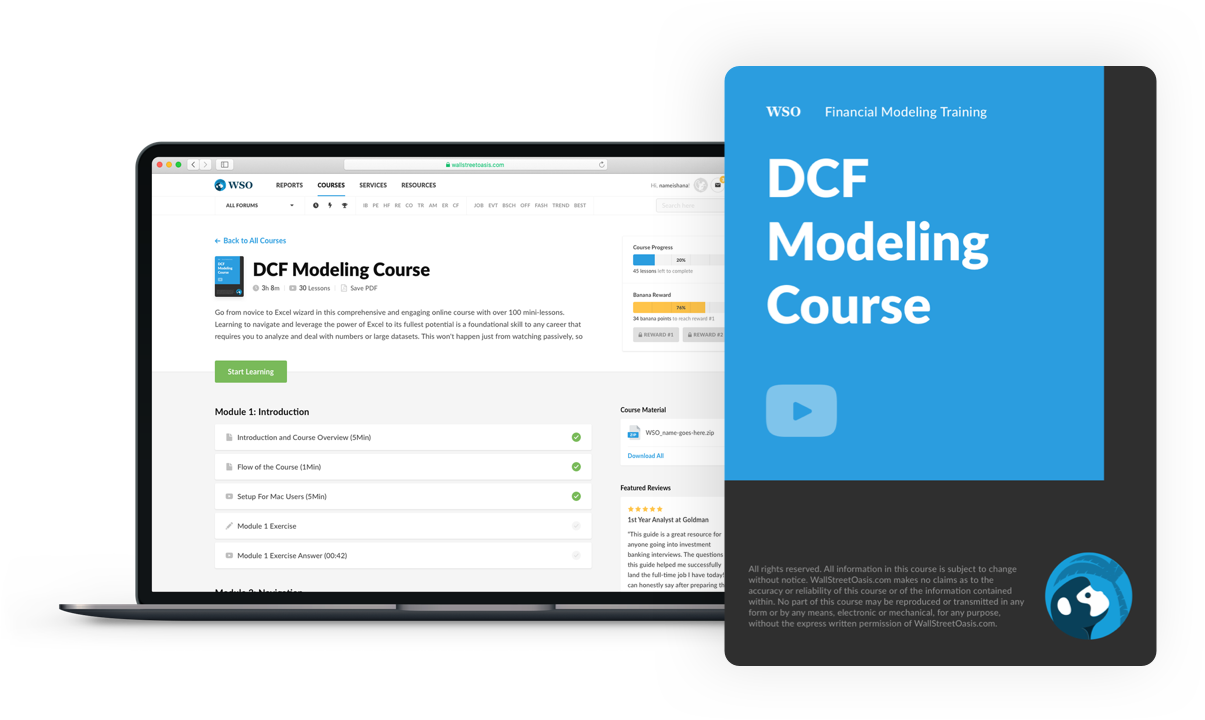### Everything You Need To Master DCF Modeling

To Help You Thrive in the Most Prestigious Jobs on Wall Street.

Researched and authored by Haimeng YangLinkedIn

Reviewed and edited by Parul GuptaLinkedIn